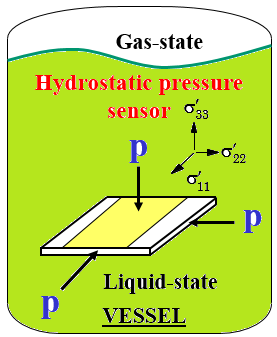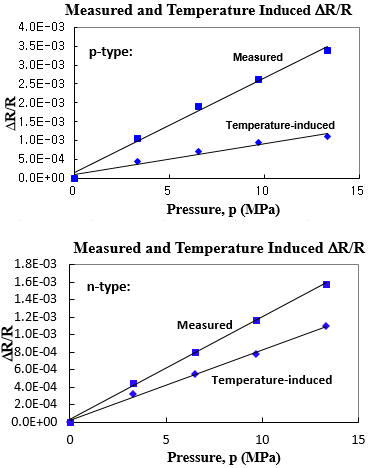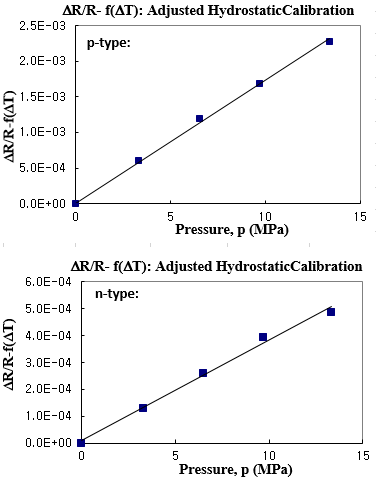Mobile QR Code1. (Department of Electronic & Electrical Engineering, College of Science and Technology, Hongik University, Sejong, 30016, Korea)
2. (School of Electronic & Electrical Engineering, College of Engineering, Hongik University, Wowsan-Ro 94, Seoul, 04066, Korea)

Semiconductor pressure-sensor, hydrostatic pressure, pressure-sensitivity

## I. INTRODUCTION

For many years, hydrostatic pressure sensors have been widely studied for stress measurements such as in the break system of a vehicle as sensing elements in various transducers[1-10]. In the future, the applications of hydrostatic pressure sensors will be widened such as in the medical field for blood pressure monitoring with development of wearable devices. In addition, hydrostatic pressure sensors can be applicable to various fields of micro-electronics or optics such as wavelength tuning of laser diodes and birefringent measurements.

For hydrostatic pressure calculation, semiconductor piezo-resistive sensors are generally used for its small size. Piezo-resistive sensors are generally composed of resistor stress sensors which are conveniently fabricated into the surface of the die using current microelectronic technology[11-15]. Expressions of resistance changes for piezo-resistive stress sensors were derived for stress measurements.

So far, a single meandering-patterned resistor has been used for hydrostatic pressure measurement. However, this method required the laborious $\Delta R/R$ measurement for an individual resistor, that is, we needed to check the change in current with respect to the sweeping voltage for resistance measurement, and what is worse it exhibited a very low sensitivity. In this work, the measuring inconveniences was resolved by calibrating the mid-point voltage of the combined (p-type in the n-substrate and n-type in the p-well) resistor sensor pair, and even the sensitivity was improved drastically by controlling the applied voltage. It should be noted that we had already shown the mid-point measurement method for normal-stress and shear-stress calculation in our previous works. Now, we extended the application to the hydrostatic pressure measurement.

## II. GENERAL THEORIES

Hydrostatic pressure is the force acting on a unit area for the object surrounded by gases or liquids. A schematic for the hydrostatic pressure-sensor is shown in Fig. 1.

Fig. 1. A schematic for the hydrostatic pressure-sensor system.We analyzed the hydrostatic pressure sensitivities of silicon for the selected silicon surfaces, resistor angle with respect to the primary axis, and sensor configurations.

### 1. (001) Silicon Surface

For the primed axes, the expression for a resistor sensor at counter-clockwise angle $\phi$ with respect to the $x_{1}^{\prime}$ axis is given by 

##### (1)
$\frac{\Delta R_{\varphi}}{R_{\varphi}}=\left[\left(\frac{\pi_{11}+\pi_{12}+\pi_{44}}{2}\right) \sigma_{11}^{\prime}+\left(\frac{\pi_{11}+\pi_{12}-\pi_{44}}{2}\right) \sigma_{22}^{\prime}\right] \cos ^{2} \varphi$

$+\left[\left(\frac{\pi_{11}+\pi_{12}-\pi_{44}}{2}\right) \sigma_{11}^{\prime}+\left(\frac{\pi_{11}+\pi_{12}+\pi_{44}}{2}\right) \sigma_{22}^{\prime}\right] \sin ^{2} \varphi$

$+\pi_{12} \sigma_{33}^{\prime}+\left(\pi_{11}-\pi_{12}\right) \sigma_{12}^{\prime} \sin 2 \varphi$

For the unprimed axes, the expression for a resistor sensor at counter-clockwise angle $\phi$ with respect to the $x_{1}$ axis is given by 

##### (2)
$\frac{\Delta R_{\varphi}}{R_{\varphi}}=\left[\pi_{11} \sigma_{11}+\pi_{12}\left(\sigma_{22}+\sigma_{33}\right)\right] \cos ^{2} \varphi+\left[\pi_{11} \sigma_{22}+\pi_{12}\left(\sigma_{11}+\sigma_{33}\right)\right] \sin ^{2} \varphi + \pi_{44} \sigma_{12} \sin 2 \varphi+\mathrm{f}(\Delta \mathrm{T})$

### 2. (111) Silicon Surface

The general expression for resistance change under stress is given by 

##### (3)
$\frac{\Delta R_{\varphi}}{R_{\varphi}}=\left[B_{1} \sigma_{11}^{\prime}+B_{2} \sigma_{22}^{\prime}+B_{3} \sigma_{33}^{\prime}-2 \sqrt{2}\left(B_{2}-B_{3}\right) \sigma_{23}^{\prime}\right] \cos ^{2} \varphi$

$+\left[B_{2} \sigma_{11}^{\prime}+B_{1} \sigma_{22}^{\prime}+B_{3} \sigma_{33}^{\prime}+2 \sqrt{2}\left(B_{2}-B_{3}\right) \sigma_{23}^{\prime} / \sin ^{2} \varphi\right.$

$+\left[2 \sqrt{2}\left(B_{3}-B_{2}\right) \sigma_{13}^{\prime}+\left(B_{1}-B_{2}\right) \sigma_{12}^{\prime}\right] \sin 2 \varphi$

where

$B_{1}=\frac{\pi_{11}+\pi_{12}+\pi_{44}}{2}$, $B_{2}=\frac{\pi_{11}+5 \pi_{12}-\pi_{44}}{6}$, $B_{3}=\frac{\pi_{11}+2 \pi_{12}-\pi_{44}}{3}$

If a sensor is subjected to hydrostatic pressure ($\sigma_{11}^{\prime}=\sigma_{22}^{\prime}=\sigma_{33}^{\prime}=-p$, $\sigma_{12}^{\prime}=\sigma_{13}^{\prime}=\sigma_{23}^{\prime}=0$) in Eq. (1) through Eq. (3), the general expression for any $\phi$ is given by

##### (4)
$\frac{\Delta R_{\varphi}}{R_{\varphi}}=-\left(\pi_{11}+2 \pi_{12}\right) p+f(\Delta T)=-\left(B_{1}+B_{2}+B_{3}\right) p+f(\Delta T) = \pi_{p} \cdot p+f(\Delta T)$

where the piezoresistive ‘pressure coefficient’ $\pi_{p}$ is defined as $-\left(\pi_{11}+2 \pi_{12}\right)=-\left(\mathrm{B}_{1}+\mathrm{B}_{2}+\mathrm{B}_{3}\right)$ and $f (\Delta T)$ denotes the temperature-induced fractional resistance by the temperature change $\Delta T$ during measurements. In Eq. (4), it is obvious that $\pi_{p}$ is regardless the same for any silicon surface, angle, and configuration, implying that any silicon conductor remains isotropic under a hydrostatic pressure. In this work, we picked 0-degree p- and n-type sensors. It should be noted that $f (\Delta T)$ affects the pressure coefficient. During the application of hydrostatic pressure, the temperature change of the hydraulic fluid is generally observed, particularly for a small volume of vessel. Therefore, the slope of temperature compensated resistance change versus applied hydrostatic pressure yields pressure coefficients for p- and n-type silicon. The expected values for the pressure coefficients are small in both p- and n-type silicon, so direct measurement of the values is quite difficult. In fact, it has been shown theoretically based on the electron-transfer mechanism in n-type silicon that $\pi_{11} \approx-2 \pi_{12}$ in n-type silicon so that $\pi_{p}$ should be very small for n-type material. Impurity concentrations affect the pressure coefficient $\pi_{p}$ is. As doping levels go up, the magnitude in pressure coefficient decreases.

## III. DESIGN & ANALYSIS

The test chip contains the p-type and n-type resistor. Resistors are often designed with relatively large meandering patterns to achieve acceptable resistance levels for measurement. In previous our works, it was observed that the pressure coefficients for both the p- and n-type silicon have small values ($\pi _{p, p}$ = 145.9/TPa (p-type) and $\pi _{p, n}$ = 31.0/TPa (n-type) at room temperature). The layout of the test chip for hydrostatic tests is shown in Fig. 2.

The voltage for an n-substrate was set to be the same as the applied voltage V (positive value), whereas the voltage for a p-well was set to be 0 (GND). This is for electrical isolation between the doped surface resistor and the bulk of the chip by using proper reverse biasing of the resistor and substrate regions. The pad ‘X’ denotes the junction of p- and n-type resistor sensors. We applied the constant voltage between pad ‘V’ (+5V) and pad ‘GND’ (0V). Now, we proposed and analyzed a measurement-method in which the hydrostatic pressure sensitivity can be determined by the change in ($R_{p}$/$R_{n}$) with respect to hydrostatic pressure p as follows:

##### (5)
$\frac{d}{d p}\left(\frac{R_{p}}{R_{n}}\right)=\frac{d}{d p}\left(\frac{x}{V-x}\right)=\frac{d x}{d p} \cdot \frac{d}{d x}\left(\frac{x}{V-x}\right) = \frac{V}{(V-x)^{2}} \cdot \frac{d x}{d p}$

where x is the junction voltage.

##### (6)
$x=V \cdot \frac{R_{p o}\left[1+\pi_{p, p} p+f(\Delta T)_{p}\right]}{R_{p o}\left[1+\pi_{p, p} p+f(\Delta T)_{p}\right]+R_{n o}\left[1+\pi_{p, n} p+f(\Delta T)_{n}\right]}$

where subscript p and n denotes p-type and n-type, respectively. Also, Rpo and Rno denotes the reference resistance value of p- and n-type during measurements, respectively.Calibration of $f ( \Delta T )$ is needed to accurately determine the pressure coefficients. Then, substitution Eq. (6) into Eq. (5) yields

##### (7)
$\frac{d}{d p}\left(\frac{R_{p}}{R_{n}}\right)=\frac{V}{(V-x)^{2}} \cdot \frac{d x}{d p}=\frac{1}{V}\left\{1+\frac{R_{p o}\left[1+\pi_{p, p} p+f(\Delta T)_{p}\right]}{R_{n o}\left[1+\pi_{p, n} p+f(\Delta T)_{n}\right]}\right\}^{2} \cdot \frac{d x}{d p}$

Also, we can express Eq. (5) as

##### (8)
$\frac{d}{d p}\left(\frac{R_{p}}{R_{n}}\right)=\frac{d}{d p}\left\{\frac{R_{p o}\left[1+\pi_{p, p} p+f(\Delta T)_{p}\right]}{R_{n o}\left[1+\pi_{p, n} p+f(\Delta T)_{n}\right]}\right\} = \frac{R_{p o}\left[1+f(\Delta T)_{p}\right]}{R_{n o}\left[1+f(\Delta T)_{n}\right]} \cdot \frac{d}{d p}\left\{\frac{1+\left(\pi_{p, p} p\right) /\left[1+f(\Delta T)_{p}\right]}{1+\left(\pi_{p, n} p\right) /\left[1+f(\Delta T)_{n}\right]}\right\}$

where $\frac{\pi_{p, p} p}{1+f(\Delta T)_{p}} << 1$ and $\frac{\pi_{p, n} p}{1+f(\Delta T)_{n}} << 1$ for any stress level. Using the approximation theory for $A << 1$ and $B << 1$, $(1+\mathrm{A}) /(1+\mathrm{B}) \approx(1+\mathrm{A})(1-\mathrm{B}) \approx 1+\mathrm{A}-\mathrm{B}$ leads to

##### (9)
$\frac{d}{d p}\left(\frac{R_{p}}{R_{n}}\right)=\frac{R_{p o}\left[1+f(\Delta T)_{p}\right]}{R_{n o}\left[1+f(\Delta T)_{n}\right]} \cdot \frac{d}{d p}\left[1+\frac{\pi_{p, p} p}{1+f(\Delta T)_{p}}-\frac{\pi_{p, n} p}{1+f(\Delta T)_{n}}\right]$

$=\frac{R_{p o}\left[1+f(\Delta T)_{p}\right]}{R_{n o}\left[1+f(\Delta T)_{n}\right]}\left[\frac{\pi_{p, p}}{1+f(\Delta T)_{p}}-\frac{\pi_{p, n}}{1+f(\Delta T)_{n}}\right]$

From Eqs. (7, 9), the hydrostatic-pressure sensitivity can be expressed as the change in x with respect to p as follows:

##### (10)
$\frac{d x}{d p}=V \frac{R_{p o}\left[1+f(\Delta T)_{p}\right]}{R_{n o}\left[1+f(\Delta T)_{n}\right]}\left\{\frac{R_{n o}\left[1+\pi_{p, n} p+f(\Delta T)_{n}\right]}{R_{p o}\left[1+\pi_{p, p} p+f(\Delta T)_{p}\right]+R_{n o}\left[1+\pi_{p, n} p+f(\Delta T)_{n}\right]}\right\}^{2} \cdot\left[\frac{\pi_{p, p}}{1+f(\Delta T)_{p}}-\frac{\pi_{p, n}}{1+f(\Delta T)_{n}}\right]$

$\cong V \frac{R_{p o}\left[1+\pi_{p, p} p+f(\Delta T)_{p}\right] \cdot R_{n o}\left[1+\pi_{p, n} p+f(\Delta T)_{n}\right]}{\left\{R_{p o}\left[1+\pi_{p, p} p+f(\Delta T)_{p}\right]+R_{n o}\left[1+\pi_{p, n} p+f(\Delta T)_{n}\right]\right\}^{2}}\left[\frac{\pi_{p, p}}{1+f(\Delta T)_{p}}-\frac{\pi_{p, n}}{1+f(\Delta T)_{n}}\right]$

$\cong V \frac{R_{p} \cdot R_{n}}{\left(R_{p}+R_{n}\right)^{2}}\left[\frac{\pi_{p, p}}{1+f(\Delta T)_{p}}-\frac{\pi_{p, n}}{1+f(\Delta T)_{n}}\right]$

where $\mathrm{R}_{\mathrm{po}}\left[1+\pi_{\mathrm{p}, \mathrm{p}} \mathrm{p}+\mathrm{f}(\Delta \mathrm{T})_{\mathrm{p}}\right]$ is the final value for p-type resistance during hydrostatic measurements ($\equiv \mathrm{R}_{\mathrm{p}}$) while $\mathrm{R}_{\mathrm{no}}\left[1+\pi_{\mathrm{p}, \mathrm{n}} \mathrm{p}+\mathrm{f}(\Delta \mathrm{T})_{\mathrm{n}}\right]$ is the final value for n-type resistance ($\equiv \mathrm{R}_{\mathrm{n}}$). Also, by approximation in Eq. (10), $\mathrm{R}_{\mathrm{po}}\left[1+\pi_{\mathrm{p}, \mathrm{p}} \mathrm{p}+\mathrm{f}(\Delta \mathrm{T})_{\mathrm{p}}\right] \approx \mathrm{R}_{\mathrm{po}}\left[1+\mathrm{f}(\Delta \mathrm{T})_{\mathrm{p}}\right]$ and $\mathrm{R}_{\mathrm{no}}\left[1+\pi_{\mathrm{p}, \mathrm{n}} \mathrm{p}+\mathrm{f}(\Delta \mathrm{T})_{\mathrm{n}}\right] \approx \mathrm{R}_{\mathrm{no}}\left[1+\mathrm{f}(\Delta \mathrm{T})_{\mathrm{n}}\right]$ because $\pi_{\mathrm{p}, \mathrm{p} \mathrm{p}} << \left(1+\mathrm{f}(\Delta \mathrm{T})_{\mathrm{p}}\right)$ and $\pi_{\mathrm{p}, \mathrm{n}} \mathrm{p} << \left(1+\mathrm{f}(\Delta \mathrm{T})_{\mathrm{n}}\right)$. Also, in Eq. (10), defining $V \cdot\left(R_{p} \cdot R_{n}\right) /\left(R_{p}+R_{n}\right)^{2} \equiv M$ leads to

##### (11)
$\frac{d x}{d p}=M \cdot\left[\frac{\pi_{p, p}}{1+f(\Delta T)_{p}}-\frac{\pi_{p, n}}{1+f(\Delta T)_{n}}\right]$

where M can be increased by controlling the applied voltage V. Also, the case in which $R_{p}$ = $R_{n}$ gives the maximum M of V/4. In our cases, $R_{p}$=10.5KΩ and $R_{n}$=2.25KΩ at room temperature. Therefore, M = 0.145·V. If we set V=20, we may have approximately 3 times magnification (M=2.90) for measurement of hydrostatic pressure.

It should be noted that ($\pi_{p, p}-\pi_{p, n}$) in Eqs. (10, 11) must be modified along with the reference temperature. Because the piezo-resistive coefficients decrease as temperature goes up, the magnitude in $\pi_{p, p}$, $\pi_{p, n}$, and therefore the magnitude in ($\pi_{p, p}-\pi_{p, n}$) also decrease with rising temperature. In order to cover the wide temperature ranges, we must have the data of $\pi_{\mathrm{p}}\left(=-\left(\pi_{11}+2 \pi_{12}\right)\right)$ for p- and n-type silicon over the temperature ranges. $\pi_{11}$ and $\pi_{12}$ for both p- and n-type can be determined by the four-point bending (4PB) technique in which only in-plane normal stress $\sigma_{11}$ (and/or $\sigma _{11}^{\prime}$) is induced. During hydrostatic tests, the die is put into the pressure vessel, whose set up is shown in Fig. 3. A pump connected to the vessel is used to generate pressure.

During the application of pressure, a change in T is inevitable. Also, for determining $\mathrm{f}(\Delta \mathrm{T})_{\mathrm{p}}$ and $\mathrm{f}(\Delta \mathrm{T})_{\mathrm{n}}$ in Eq. (10), TCR (temperature coefficient of resistance) measurements are required. $\mathrm{f}(\Delta \mathrm{T})_{\mathrm{p}}$ and $\mathrm{f}(\Delta \mathrm{T})_{\mathrm{n}}$ may be extracted by measuring the normalized resistance change with respect to a temperature change. To this purpose, extensive calibrations of resistance with varying temperatures for p- and n-type are performed in a temperature controllable chamber. During the TCR measurements, no stress is applied. For high temperatures, we used a resistance heater inside the pressure vessel to increase the temperature of fluid. To lower the temperature of fluid, we used liquid nitrogen, which is injected into a specially designed box surrounding the pressure vessel. The recording of the actual temperature is made by a thermistor inside the vessel. At a given reference temperature, a quadratic equation is enough to fit $\mathrm{f}(\Delta \mathrm{T})$ over any small range of temperature, especially for the hydrostatic tests, for both p-type and n-type samples. However, if the temperature is assumed to be maintained constant at the reference temperature ($\mathrm{f}(\Delta \mathrm{T})$=0) during the measurement, Eq. (10) reduces to

##### (12)
$\frac{d x}{d p}=V \cdot \frac{R_{p} \cdot R_{n}}{\left(R_{p}+R_{n}\right)^{2}}\left(\pi_{p, p}-\pi_{p, n}\right)$

The comparisons of hydrostatic-pressure sensitivity between previous works and our newly proposed works are shown in Table 1.

Table 1. Comparison of hydrostatic-pressure sensitivity between the ‘conventional’ method and ‘combined’ method

 Method Sensitivity Expression I. Con. (p-) $\pi_{\mathrm{p}, \mathrm{p}}$ $\Delta \mathrm{R} / \mathrm{R}=\pi_{\mathrm{p}, \mathrm{p}} \cdot \mathrm{p}+\mathrm{f}(\Delta \mathrm{T})_{\mathrm{p}}$ II. Con. (n-) $\pi_{\mathrm{p}, \mathrm{n}}$ $\Delta \mathrm{R} / \mathrm{R}=\pi_{\mathrm{p}, \mathrm{n}} \cdot \mathrm{p}+\mathrm{f}(\Delta \mathrm{T})_{\mathrm{n}}$ III. Combined (p- & n-) $\mathrm{M} \cdot\left(\pi_{\mathrm{p}, \mathrm{p}} /\left[1+\mathrm{f}(\Delta \mathrm{T})_{\mathrm{p}}\right]-\right.$ $\pi_{\mathrm{p}, \mathrm{n}} /\left[1+\mathrm{f}(\Delta \mathrm{T})_{\mathrm{n}}\right] )$ $\mathrm{d} \mathrm{x} / \mathrm{dp}=\mathrm{M} \cdot\left(\pi_{\mathrm{p}, \mathrm{p}} /\left[1+\mathrm{f}(\Delta \mathrm{T})_{\mathrm{p}}\right]-\right.$$\pi_{\mathrm{p,n}} /\left[1+\mathrm{f}(\Delta \mathrm{T})_{\mathrm{n}}\right] )$

In our previous works, we evaluated the pressure coefficient by performing the hydrostatic experiments. By subtraction of the temperature-induced resistance change $\mathrm{f}(\Delta \mathrm{T})$, adjusted pressure coefficient for p- and n-type sensors were obtained ($\pi _{p, p}$ = 145.9 TPa-1, $\pi _{p, n}$ = 31.0 TPa-1). At room temperature, a linear (or quadratic) equation is enough to fit $\mathrm{f}(\Delta \mathrm{T})$ over any small range of temperature, especially for the hydrostatic tests, for both p-type and n-type samples. Around the room temperature with stress-free conditions, $\mathrm{f}(\Delta \mathrm{T})_{p}$ = 1.56·10-3 $\Delta T$/℃ and $\mathrm{f}(\Delta \mathrm{T})_{n}$ = 1.70·10-3 $\Delta T$/℃. During the application of hydrostatic pressure, p up to 13.3 MPa, the temperature change of the hydraulic fluid was about 0.67℃. An example of measured and temperature-induced normalized resistance change are plotted together for p- and n-type resistor sensor (see Fig. 4). Also, adjusted resistance change for p- and n-type resistor sensor is presented in Fig. 5.

Fig. 4. An example of $\Delta R/R$ for p- and n-type resistors during hydrostatic test.Fig. 5. Adjusted resistance change for p- and n-type resistors during hydrostatic test.If we assume the same conditions as above (hydrostatic pressure, p = 13.3 MPa, $\Delta T$ = 0.67 ℃), the hydrostatic pressure sensitivity ‘dx/dp’ in Eq. (11) is calculated in Table 2.

Table 2. Analysis in hydrostatic-pressure sensitivity in our proposed method

 V [v] M $\pi_{p, p} /\left[1+\mathrm{f}(\Delta \mathrm{T})_{\mathrm{p}}\right]-\pi_{\mathrm{p,n}} /\left[1+\mathrm{f}(\Delta \mathrm{T})_{\mathrm{n}}\right]$ [TPa-1] dp/dx [TPa-1] 5 0.725 114.78 83.2 10 1.450 114.78 166.4 15 2.175 114.78 249.6 20 2.900 114.78 332.8

Compared to p-type sensor, ($\pi_{p, p}$ = 145.9 TPa-1), this proposed method roughly enhanced the hydrostatic-pressure sensitivity by 15% for V = 10 and 130% for V=20. The sensitivity comparisons among the previous works (p-type and n-type) and the proposed method (p- and n-type combined) are shown in Fig. 6.

## IV. CONCLUSIONS

For the accurate hydrostatic pressure calculation, we derived the mathematical and analytical expressions in which a temperature change during measurements was considered. In this work, we could achieve the high hydrostatic-pressure sensitivity, compared to the previous results of our works, by controlling the applied voltage V. Here, we exploited the sensitivity difference between p- and n-type sensors. And then, we amplified its difference by increasing the applied voltage V. We just needed to measure the junctional mid-point voltage ‘x’ in p- and n-type combined semiconductor resistor sensors. So far, for the measurement of the hydrostatic-pressure, we have used one type single resistor sensor whose resistance change is very difficult to measure due to its low sensitivity. Also, we analyzed the sensitivity in hydrostatic-pressure between conventional method and our newly proposed method. The magnitude in sensitivity of the mid-point voltage with respect to hydrostatic-pressure, p, was approximately increased by 14% for V = 10 (128% for V =20).

### ACKNOWLEDGMENTS

This work was supported by 2019 Hongik University Research Fund, and this work was supported by Basic Science Research Program through the Ministry of Education of the Republic of Korea and National Research Foundation of Korea (2016R1D1A1B03935561).

### REFERENCES

1
Templeton M., 1982, Fermi-surface anisotropy in Potassium and the Effects of Hydrostatic Pressure, J. of Low Temp. Phys., Vol. 47, pp. 187-1872
Suhling J. C., Jaeger R. C., 2001, Silicon Piezoresistive Stress Sensors and Their Application in Electronic Packaging, IEEE Sensors J., Vol. 1, No. 1, pp. 14-303
Cho C. H., et al , 2008, Characterization of the Temperature Dependence of the Piezoresistive Coefficients of Silicon From -150℃ to +125℃, IEEE Sensors J., Vol. 8, No. 8, pp. 1455-14684
Cho C. H., et al , 2008, Evaluation of the Temperature Dependence of the Combined Piezoresistive Coefficients of (111) Silicon Utilizing Chip-on-Beam and Hydrostatic Calibration, JKPS, Vol. 52, pp. 612-6205
Li Z., et al , 2015, An Improved Method for the Mechanical Behavior Analysis of Electrostatically Actuated Microplates Under Uniform Hydrostatic Pressure, J. Micro-electro-mechanical Sys., Vol. 24, No. 2, pp. 474-4856
Domingues M., et al , 2015, Liquid Hydrostatic Pressure Optical Sensor Based on Micro-Cavity Produced by the Catastrophic Fuse Effect, IEEE Sensors J., Vol. 15, No. 10, pp. 5654-56587
Ayyanar N., et al , 2017, Hydrostatic Pressure Sensor Using High Birefringence Photonic Crystal Fibers, IEEE Sensors J., Vol. 17, No. 3, pp. 650-6568
Patel M., Sinha B., 2018, A Dual-Mode Thickness-Shear Quartz Pressure Sensor for High Pressure Applications, IEEE Sensors J., Vol. 18, No. 12, pp. 4893-49019
El-Yadri M., et al , 2018, Temperature and Hydrostatic Pressure Effects on Single Dopant States in Hollow Cylindrical Core-shell Quantum Dot, App. Surface Sci., Vol. 441, pp. 204-20910
Wei J., Zhang Z., et al , 2018, Effects of High Hydrostatic Pressure on Structural and Physical Properties of Nisin-SPI Film, International J. of Biological Macromolecules, Vol. 111, pp. 976-98211
Morin F. J., et al , 1956, Temperature Dependence of the Piezoresistance of High-Purity Silicon and Germanium, Phys. Rev., Vol. 10, No. 2, pp. 525-53912
Jaeger R. C., Suhling J. C., 1993, Thermally induced Errors in the Calibration and Application of Silicon Piezoresistive Stress Sensors, Advances in Electronic Packaging, ASME, EEP, Vol. 4, No. 1, pp. 457-47013
Lund E., Finstad T., 1999, Measurement of the Temperature Dependency of the Piezoresistance Coefficients in p-type Silicon, Advances in Electronic Packaging, Vol. 1, pp. 215-21814
Richter J., Hansen O., 2005, Piezoresistance of Silicon and Strained Si0.9Ge0.1, Sensors and Actuators A, Vol. 123-124, pp. 388-39615
Cho C. H., et al , 2017, A Study on Shear-Stress Calibration by the Mid-Point Measurements in +45/-45 Degree Semiconductor Resistor-Pair, JSTS, Vol. 7, No. 2, pp. 180## Author

received the B.S. degree in Electrical Engineering from the Seoul National University, Seoul, South Korea, in 1997, and the M.S. and Ph.D. degrees in Electrical and Computer Engineering from Auburn University, Auburn, AL, in 2001 and 2007, respectively.

In 2009, he joined Hongik University, Sejong where he is currently an Associated professor in the Department of Electronic & Electrical engineering.

His research interests include the application of analytical and experimental methods of piezoresistive sensors to problems in electronic packaging.

received the B.S. and M.S. degrees in electrical engineering from from Seoul National University, Seoul, Korea, in 1996 and 1999, respectively, and the Ph.D. degree in electrical and computer engineering from Cornell University, Ithaca, NY, in 2004.

He was a Postdoctoral Research Associate with Cornell University until 2005, where he focused on the design and fabrication of SiC and GaN electronic devices and GaN nanowires.

He was with the General Electric Global Research Center, Niskayuna, NY, from 2005 to 2007, developing wide-bandgap semiconductor sensors and high-power devices.

Since 2007, he has been with Hongik University, Seoul, where he is currently a Professor in the School of Electronic and Electrical Engineering.

His research interests include wide bandgap semiconductor devices.

He has authored over 60 publications in his research area.

received the B.S. and M.S. degrees in electrical and electronic engineering from Yonsei University, Seoul, Korea, in 1999 and 2001, respectively, and Ph.D. degree in electrical engineering and computer sciences from the University of California, Berkeley, in 2006.

He was a Postdoctoral Researcher with the University of California, Berkeley.

He is now with the School of Electronic and Electrical Engineering, Hongik University, Seoul, Korea.

His research interests are in the area of optoelectronic devices, optical injection locking of semiconductor lasers, and optoelectronic oscillators.